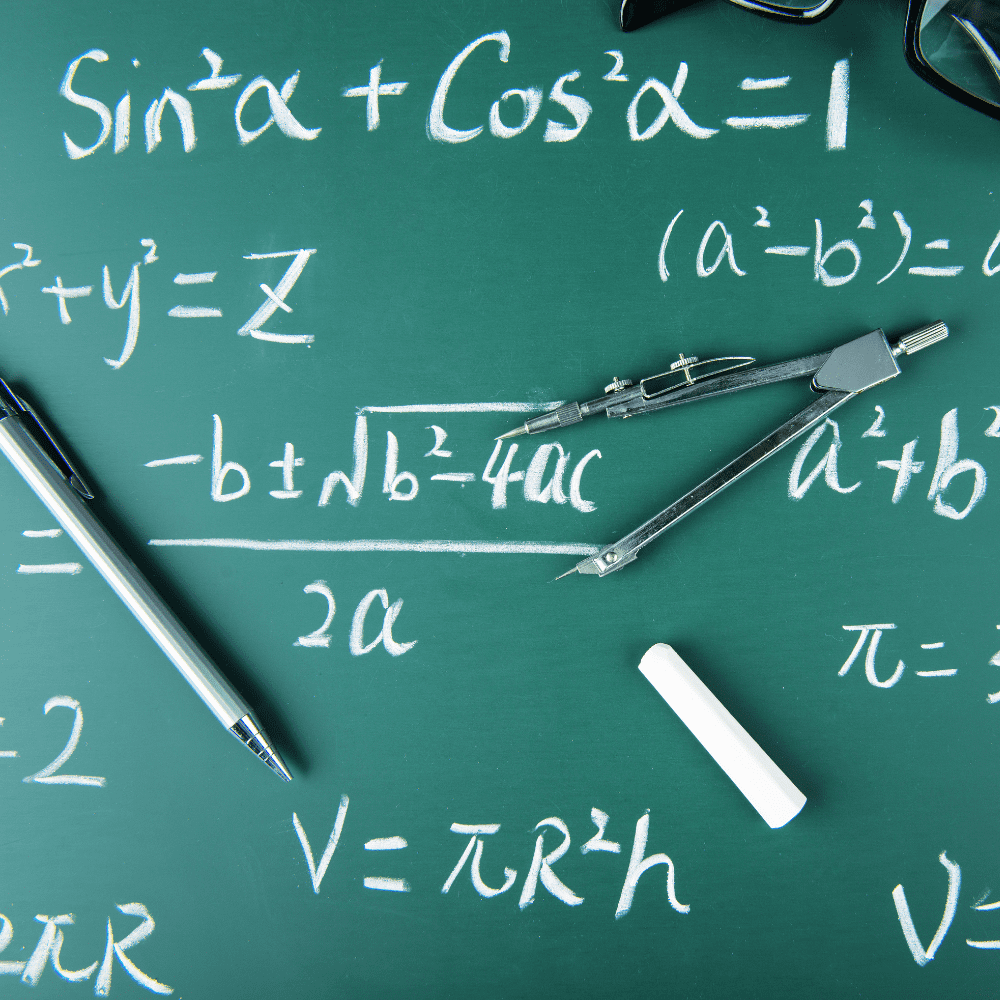# A-Level Maths Tuition

Make sure you’re prepared for the A-Level Maths Exam with TigerCampus Singapore’s private tuition today!## Overview

### Customised curriculum

We'll find a tutor who can make sure you're prepared for the A-Level Maths exam with customisable lessons catered to fit your needs.

### Flexible

Take lessons only when you need them—as little or as many as necessary until you feel confident.

### Private lesson

No need to accommodate other students. Learning is customised your perfect pace and difficulty so you are always improving.

H1 Mathematics provides students with a foundation in mathematics and statistics that will support their university studies in business or social sciences. It is especially suitable for students who do not have O-Level Additional Mathematics because it allows them to learn important mathematical concepts and skills in algebra and calculus that were taught in Additional Mathematics. Students will also learn the fundamental statistical methods required for business and social science studies.

H2 Mathematics is intended to prepare students for a variety of university courses, including mathematics, sciences, engineering, and related courses that require a strong mathematical foundation. It fosters mathematical thinking and reasoning skills, which are required for further mathematics education. Students gain an appreciation for mathematics and its connections to other disciplines and the real world through its applications.

## Description

In this course, together with a private tutor, you will learn how to master the A-Level maths & further maths exam content. Your tutor will help you understand the relevant concepts and formulas while assessing your progress through practice exams.

Unlike other A-Level Maths courses, this course is a private one-on-one lesson. You will study at the perfect pace, as opposed to being limited by your peers. You can expect to be sufficiently challenged and constantly improving, rather than wasting time reviewing concepts you already know.

• Higher 1
• Higher 2

## Topics in syllabuses

##### H1 Mathematics
###### Section A: Pure Mathematics
• Functions and Graphs
• Exponential logarithmic functions and Graphing techniques
• Equations and inequalities
• Calculus
• Differentiation
• Integration
###### Section B: Probability and Statistics
• Probability
• Binomial distribution
• Normal distribution
• Sampling
• Hypothesis testing
• Correlation and Linear regression
##### H2 Mathematics
###### Section A: Pure Mathematics
• Algebra and Calculus
• Mathematical Induction
• Complex numbers
• Polar Coordinates
• Conic sections
• Applications of definite integrals
• Differential equations
• Discrete Mathematics, Matrices and Numerical Methods
• Recurrence relations
• Matrices and linear spaces
• Numerical methods
###### Section B: Probability and Statistics
• Discrete random variables
• Continuous random variables
• Hypothesis testing and Confidence intervals
• Non-parametric tests

#### Our tutors graduated from top universities## How it works

### 1Request a tutor

Let us know your goals and age range. We'll figure out a plan to help get you there.

### 2Match with a tutor

You'll get a tutor based on your needs and goals, or you can request a specific tutor.

### 3Start a free trial

Experience a free trial lesson with your new tutor and see if your learning style matches.

### 4Keep it up!

If everything went well, sign up to keep going! You can choose the pacing of the lessons

### 1Request a tutor

Let us know your goals and age range. We'll figure out a plan to help get you there.

### 2Match with a tutor

You'll get a tutor based on your needs and goals, or you can request a specific tutor.

### 3Start a free trial

Experience a free trial lesson with your new tutor and see if your learning style matches.

### 4Keep it up!

If everything went well, sign up to keep going! You can choose the pacing of the lessons